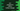# C++ program to print a hollow triangle using star# Introduction :

In this tutorial, we will learn how to print hollow triangle in C++. Our program will take the height of the triangle as an input from the user and print it out.

``````      *
* *
*   *
*     *
*       *
*         *
*************``````

This is a triangle with height 7.

I will show you one easier way to solve these types of problems. Let’s take a look:

## Designing the algorithm :

You can solve any pattern by drawing it on a paper. For the above triangle, we are filling the vacant space with blank spaces. Or we need to print blank space for the empty spaces and star * for the triangle.

Think it like you are printing the triangle on a graph paper. It will look as like below if you replace the blank space with \$ :

``````\$\$\$\$\$\$*
\$\$\$\$\$*\$*
\$\$\$\$*\$\$\$*
\$\$\$*\$\$\$\$\$*
\$\$*\$\$\$\$\$\$\$*
\$*\$\$\$\$\$\$\$\$\$*
*************``````

If we replace each \$ with a blank space, it will create the below triangle :

``````      *
* *
*   *
*     *
*       *
*         *
*************``````

This is the hollow triangle that we want to create programmatically. Now, forget about it. Let’s analyze the first triangle :

``````1st line : 6\$ => 1 (one *)
2nd line : 5\$ => 3 (first and the last is *, middle is \$)
3rd line : 4\$ => 5 (same as above)
4th line : 3\$ => 7 (same as above)
5th line : 2\$ => 9 (same as above)
6th line : 1\$ => 11 (same as above)
7th line : 0\$ => 13 (all are *)``````

Here,

• The number of \$ is kept decreasing from 6 to 0.
• The first and the last line will print only *
• After \$ is printed, other characters are printed for 2*n - 1 time, where n is the step number.
• After \$ is printed, all middle lines will print one * at the start and end with \$ in the mid.

## C++ program :

Let’s write down the above logic in the code. We will print one space instead of a \$ here :

``````#include <iostream>
using namespace std;

int main()
{
int height;

//1
cout << "Enter the height of the triangle : "; cin >> height;

//2
for (int i = 1; i <= height; i++)
{
//3
for (int j = 1; j <= (height - i); j++)
{
cout << " ";
}

//4
for (int k = 1; k <= i * 2 - 1; k++)
{
//5
if (k == 1 || k == i * 2 - 1)
{
cout << "*";
}
else if (i == height)
{
//6
cout << "*";
}
else
{
//7
cout << " ";
}

}
//8
cout << endl;
}
return 0;
}``````

## Explanation :

The commented numbers in the above program denote the step numbers below :

1. Ask the user to enter the height of the triangle. Read and store it in the height variable.
2. This will run the for loop for height number of times. This loop is used to print each row of the triangle.
3. This is an inner for loop. This loop is used to print the starting blank spaces. In the above example, it is meant for the starting \$ symbols.
4. This loop is used to print the other characters after the blank spaces. It will run from 1 to i*2 -1.
5. Print * at the start and end.
6. Print only * for the last line.
7. Else, print blank space.
8. At the end of the outer for loop, print one new line, i.e. move to the next row.

## Sample Output :

### Conclusion :

You can easily solve any print puzzle questions if you divide the problem into steps as I have shown above. Try to solve it on your own and if you have any questions, drop one comment below.